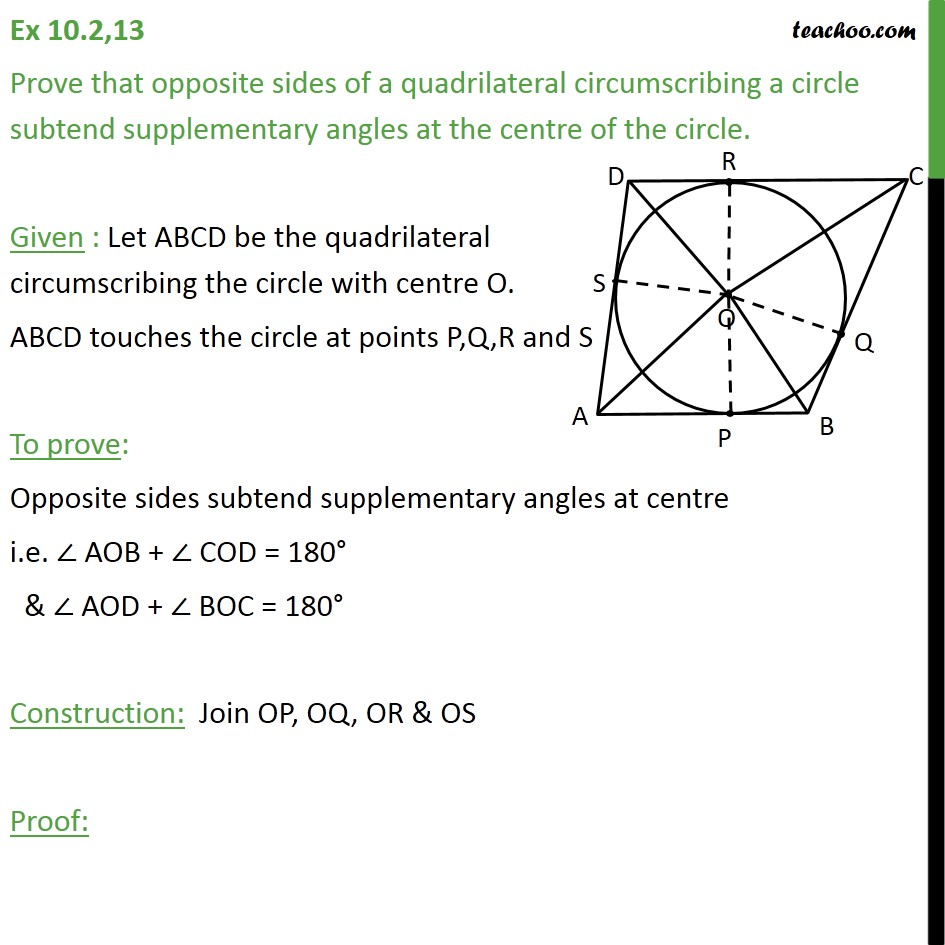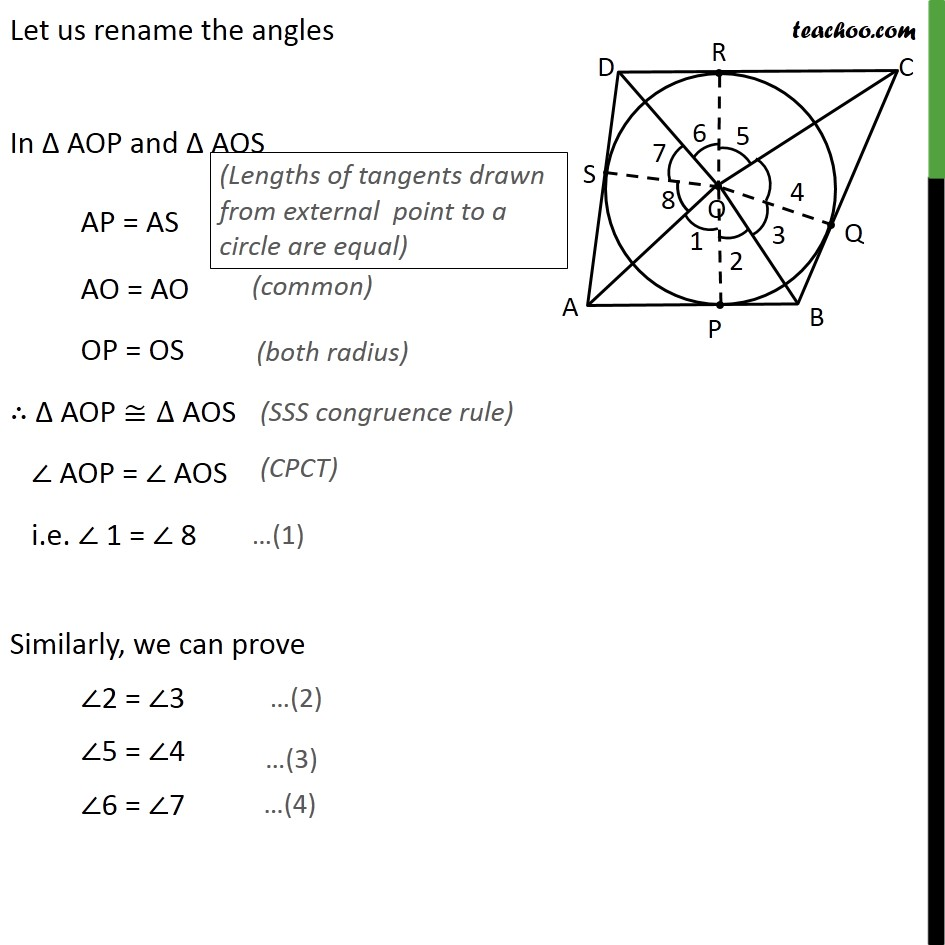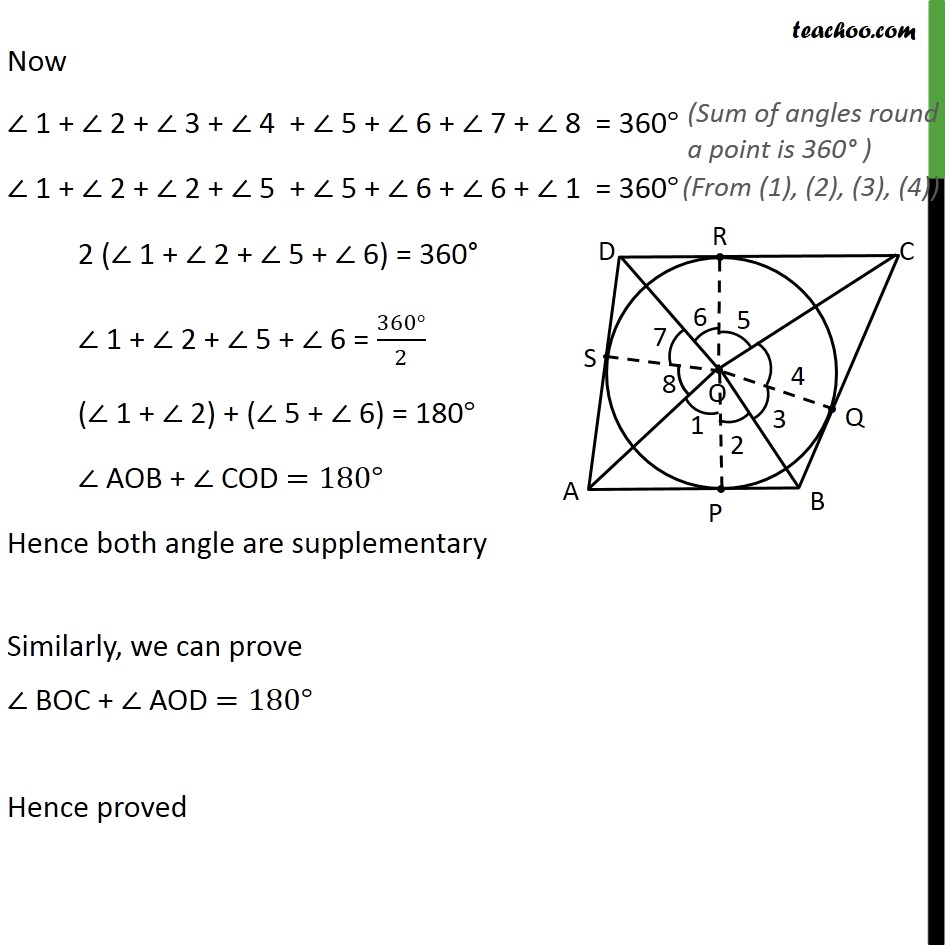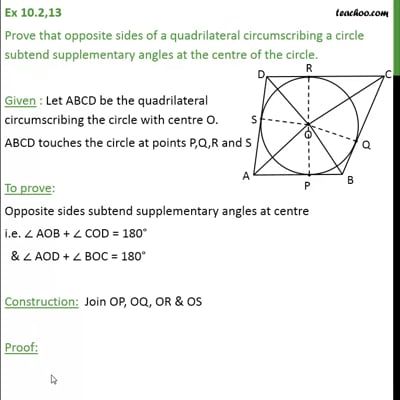Ex 10.2

Chapter 10 Class 10 Circles
Serial order wiseThis video is only available for Teachoo black users

Solve all your doubts with Teachoo Black (new monthly pack available now!)

### Transcript

Ex 10.2,13 Prove that opposite sides of a quadrilateral circumscribing a circle subtend supplementary angles at the centre of the circle. Given : Let ABCD be the quadrilateral circumscribing the circle with centre O. ABCD touches the circle at points P,Q,R and S To prove: Opposite sides subtend supplementary angles at centre i.e. ∠ AOB + ∠ COD = 180° & ∠ AOD + ∠ BOC = 180° Construction: Join OP, OQ, OR & OS Proof: Let us rename the angles In Δ AOP and Δ AOS AP = AS AO = AO OP = OS ∴ Δ AOP ≅∆ AOS ∠ AOP = ∠ AOS i.e. ∠ 1 = ∠ 8 Similarly, we can prove ∠2 = ∠3 ∠5 = ∠4 ∠6 = ∠7 Now ∠ 1 + ∠ 2 + ∠ 3 + ∠ 4 + ∠ 5 + ∠ 6 + ∠ 7 + ∠ 8 = 360° ∠ 1 + ∠ 2 + ∠ 2 + ∠ 5 + ∠ 5 + ∠ 6 + ∠ 6 + ∠ 1 = 360° 2 (∠ 1 + ∠ 2 + ∠ 5 + ∠ 6) = 360° ∠ 1 + ∠ 2 + ∠ 5 + ∠ 6 = (360°)/2 (∠ 1 + ∠ 2) + (∠ 5 + ∠ 6) = 180° ∠ AOB + ∠ COD =180° Hence both angle are supplementary Similarly, we can prove ∠ BOC + ∠ AOD =180° Hence proved